﻿ Python資料視覺化呈現，實作機器學習方法

# ntuccepaper2019

## 專題報導

### 手機版選單

#### 字級

Python資料視覺化呈現，實作機器學習方法
• 卷期：v0041
• 出版日期：2017-06-20

### Windows packages(引用官網的介紹)

Windows does not have any package manager analogous to that in Linux, so installing one of the scientific Python distributions mentioned above is preferred. However, if that is not an option, Christoph Gohlke provides pre-built Windows installers for many Python packages, including all of the core SciPy stack, which work extremely well.

### 安裝環境

1. Windows 7 64-bit (Windows 10 方法一樣)
2. Anaconda 4.3.1 for Windows
3. Python 3.6
4. TensorFlow CPU only

1. 安裝Anaconda：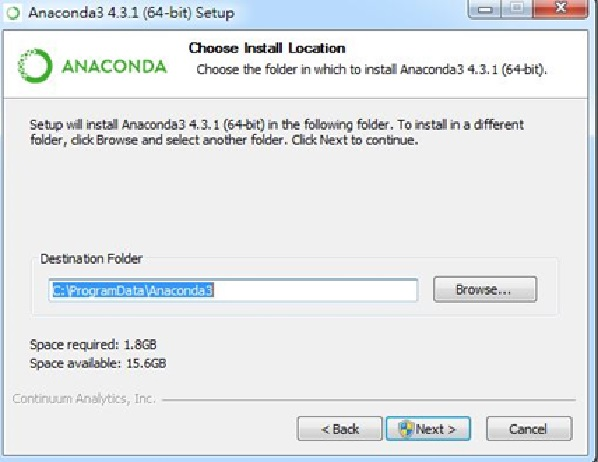Conda -V
Python -V2. 建立Python虛擬環境：

conda create --name py-keras python=3.5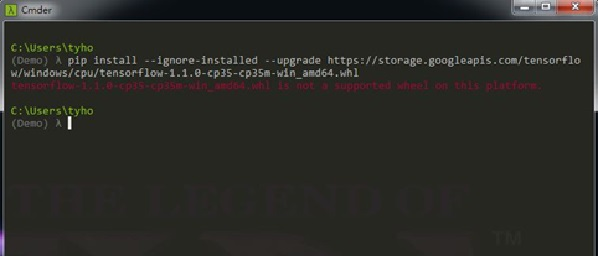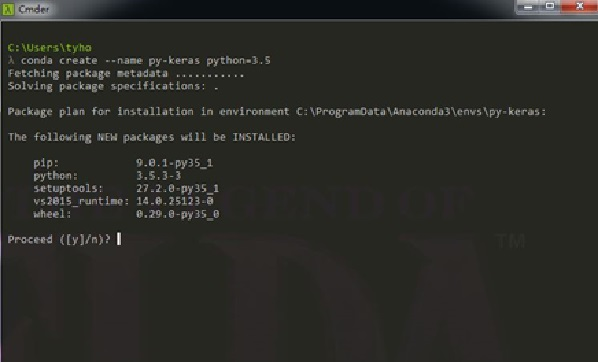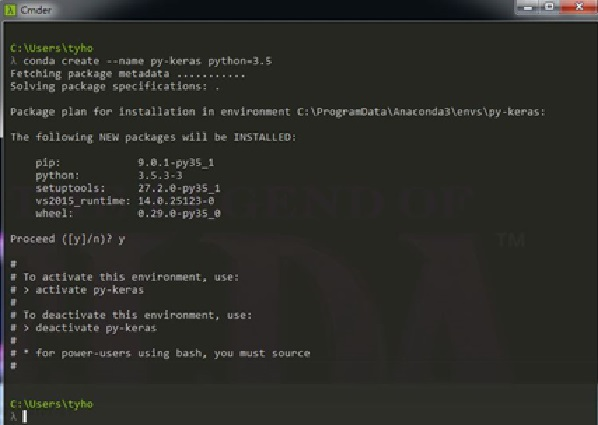activate py-keras
# deactivate py-keras 離開環境

3. 安裝Python 套件：

conda install scipy
conda install theano
conda install scikit-learn
conda install matplotlib

conda install tensorflow keras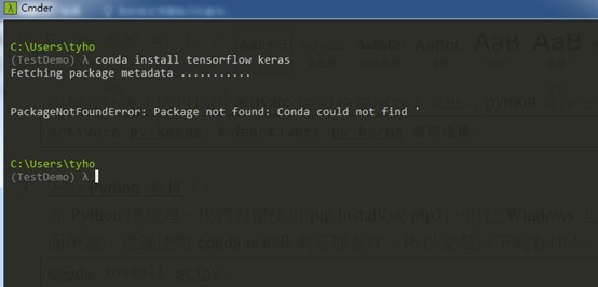pip install git+git://github.com/fchollet/keras.git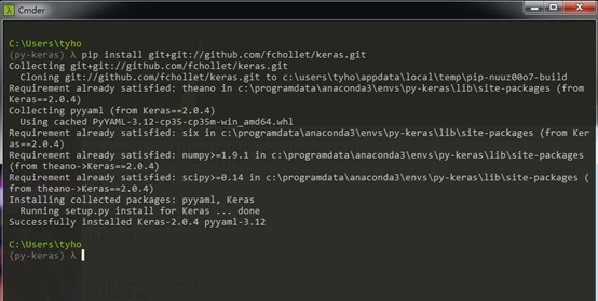4. 開啟Jupyter Notebook： 為了讓更便利將資料視覺化呈現，所以接下來的範例討論採用了Jupyter notebook來呈現(或用iPython notebook)，所以安裝jupyter如下，並開啟notebook：
conda install jupyter
jupyter notebook #開啟notebook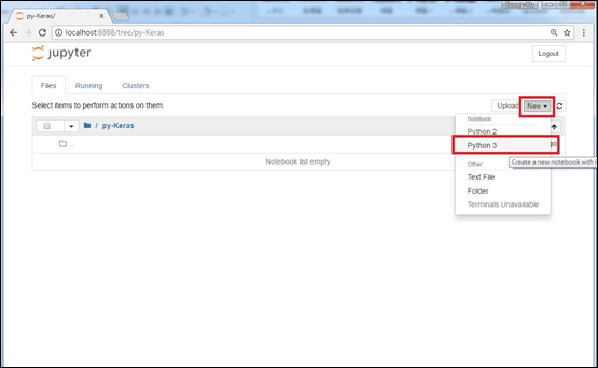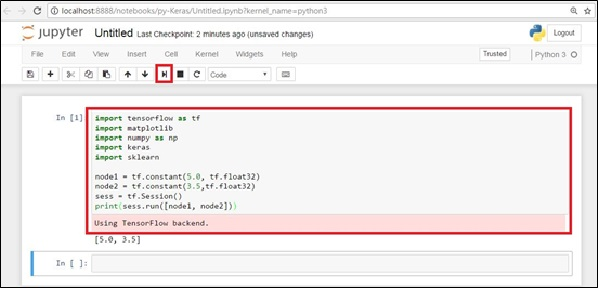import tensorflow as tf
import matplotlib
import numpy as np
import keras
import sklearn

node1 = tf.constant(5.0, tf.float32)
node2 = tf.constant(3.5,tf.float32)
sess = tf.Session()
print(sess.run([node1, node2]))

### 三、Matplotlib 資料視覺化的呈現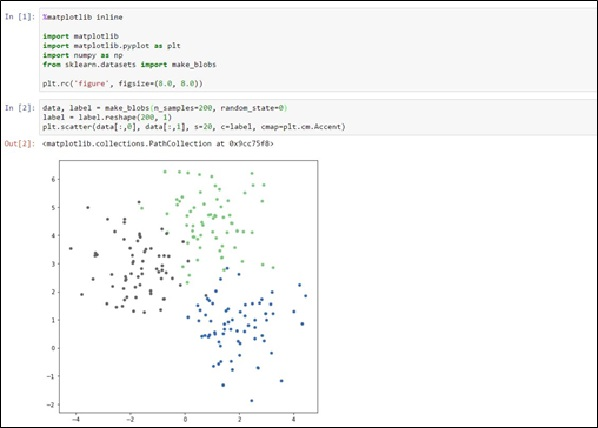%matplotlib inline

import matplotlib
import matplotlib.pyplot as plt
import numpy as np
from sklearn.datasets import make_blobs

plt.rc('figure', figsize=(8.0, 8.0))

data, label = make_blobs(n_samples=200, random_state=0)
label = label.reshape(200, 1)
plt.scatter(data[:,0], data[:,1], s=20, c=label, cmap=plt.cm.Accent)

(1) 使用matplotlib.pyplot進行畫圖，使用plt.rc定義圖片大小。
(2) 利用sklearn.datasets載入資料，本範例使用make_blobs，或用circle， API的使用可以參考，make_blobs和make_circle。
(3) n_samples 取的點數。
(4) reshape(200,1) 將200個點的陣列轉成向量，其中label的值為0或是1，在圖形上顯示兩種不同的顏色。
(5) data 為200x2的矩陣，其中data[: ,0] 表示X座標值，data[: ,1]為Y座標值，Color使用label來區分，s為資料的圖形大小。Cmap則是color map的方式。

from sklearn.datasets import make_circles
data, label = make_circles(n_samples=200, random_state=0, factor=0.8, noise=0.1)
label = label.reshape(200, 1)
plt.scatter(data[:,0], data[:,1], s=40, c=label, cmap=plt.cm.Accent)### 四、TensorFlow 介紹

TensorFlow的介紹參考https://www.tensorflow.org/get_started/get_started，本文將以這篇介紹TensorFlow，介紹核心概念。
TensorFlow的開發概念是來類神經網路，利用多個結點組合成一個網路集合，有興趣的可以自行上網搜尋相關文章。所以對TensorFlow來說，其tensor就是一個最小的神經元，也可看成一個網路node，這個node可以輸入、運算、且輸出運算結果。所以TensorFlow的Model其實就只是作三件，定義1)各個node功能，2)各node間的連結方式，3)輸入、輸出的維度。然後有了Model之後在開始train。import tensorflow as tf

x1 = tf.constant(1.0, tf.float32)
x2 = tf.constant(2.0, tf.float32)
x3 = tf.constant(3.0, tf.float32)

a = tf.placeholder(tf.float32)
b = tf.placeholder(tf.float32)

node2 = a + b * x3 + node1

sess = tf.Session()
init = tf.global_variables_initializer()
sess.run(init)
sess.run(node2, {a: 10.0, b:3.0})

(2) node1 是 x1和x2的相加。
(3) a，b是可以調變的變數，注意到這邊，一旦我們定義了變數，我們就必須先初始化變數。
(4) 最後使用session.run執行一輪，即結束。

Linear Model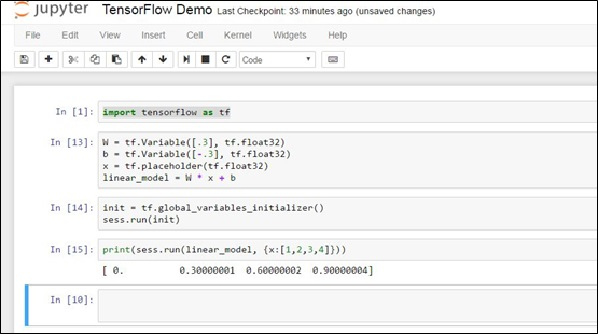### 五、Keras 介紹

from keras.models import Sequential
from keras.layers import Dense

model = Sequential()
model.compile(optimizer='sgd')
model.summary()

from keras.models import Sequential
from keras.layers import Dense

model = Sequential()
model.compile(optimizer='sgd', loss='binary_crossentropy')
model.summary()

### 六、利用Keras進行Model訓練。

https://github.com/nailo2c/PyCon2017TutorialRL/blob/master/MLP_Keras.ipynb 。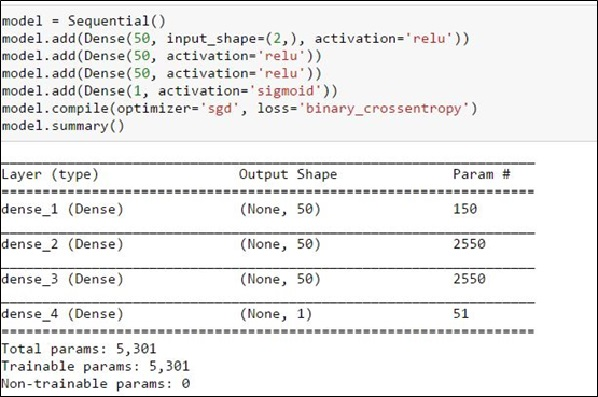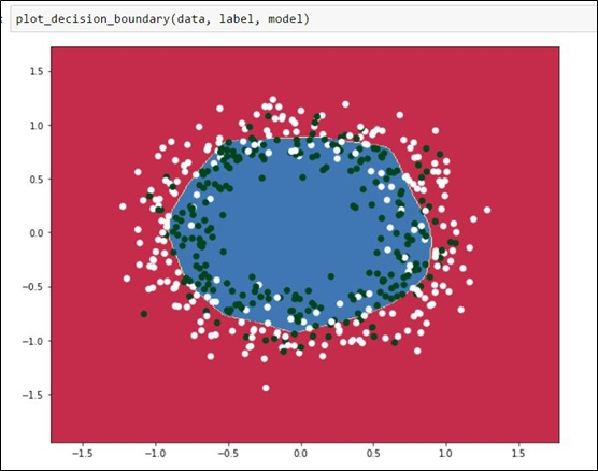### 參考資料

 Anaconda 官網
 Anaconda環境管理
https://conda.io/docs/using/envs.html
TensorFlow for Windows
https://www.tensorflow.org/install/install_windows
 Jupyter Notebook